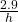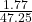## A pole that is 2.9 m tall casts a shadow that is 1.77 m long. At the same time, a nearby tower casts a shadow that is 47.25 m long. Ho

Question

A pole that is 2.9 m tall casts a shadow that is 1.77 m long. At the same time, a nearby
tower casts a shadow that is 47.25 m long. How tall is the tower? Round your answer to the nearest meter.

in progress 0
3 weeks 2021-08-25T02:43:41+00:00 1 Answers 0 views 0

77 m

Step-by-step explanation:

The 2 situations are similar , so the ratios of corresponding sides are equal.

let h be the height of the tower, then=( cross- multiply )

1.77h = 137.025 ( divide both sides by 1.77 )

h ≈ 77 m ( to the nearest metre )